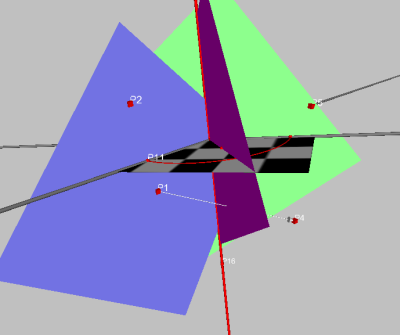#### 18.1 The in-sphere of a tetrahedron

In2 the intersection of the angle bisectors of a triangle is the midpoint of the so-called in-circle. This circle has all segments of the triangle as tangents.There is an analogous construction in3: You can construct the in-sphere of a (irregular) tetrahedron.

Conclusion by analogy shows that the intersection of three angle-bisector-planes is the midpoint of the in-sphere.

The first step of constructing the in-sphere will be the development of an angle-bisector-macro.

Unfortunately it is impossible to construct a macro ’Angle bisector’ with two planes as given, as it would be undetermined which angle-bisector should be constructed. Thus it will be necessary to define the orientation of the scene through the given objects.

This can be achieved by giving five points instead of two planes: Two points on the first plane, one shared point and two points on the second plane.

##### 18.1.1 The angle-bisector macro

If you want to use the predefined angle-bisector-macro, you can skip this paragraph.

I have used the following construction. Let P1, P2, P3, P3 and P5 be free points. PLA1 is the plane through P1, P2 and P3. PLA2 is the plane through P3, P4 and P5. PLA1 should lie ’left’, PLA2 ’right’, P1 under P2 and P4 under P5.This ensures that if you play the macro later on and use the same orientation, the angle-bisector will be the same, too.

Furthermore the macro should be as ’stable’ as possible. First we need the intersection line of the two planes.

• Construct PLA1 through P1, P2 and P3
• Construct PLA2 through P3, P4 and P5
• Construct L1 as intersection of PLA1 and PLA2Now we need a point that is exactly between the two planes, i.e. on the angle-bisector. For this we need a circle of arbitrary radius an a midpoint on the intersection-line. This could result in the angle-bisector-plane being displayed anywhere between the two planes. To avoid this, we take P3, which lies on the intersection-line, as midpoint and P1 as a point on the circle. As circle-plane we take the plane through P3 perpendicular to L1.Now we have to do the following:

• construct the intersections of the circle with the two planes (e.g. with the intersect-button)
• Construct the midpoint of the two visible intersections.
• Now we need a third point on the angle-bisector plane. This could be another arbitrary point on L1. But an arbitrary point might be very close to P3, which could make the plane ’unstable’. Thus it is better to construct a point that is definitely not close to P3. You can use a sphere with midpoint P3 and radius |P1P4|, but there are other possibilities, too.
• Now we can draw the angle-bisector-pane.Drag the base-points to see if the construction is ’stable’.

Moreover we can see what happens, if P1 is not under but over P2: The angle bisector goes ’behind’, as the orientation of the scene is reversed.

Finishing the construction all unnecessary objects (meaning all objects save the angle-bisector) should be hidden and points P1 to P5 marked as given. Do not forget to save the construction, as ’angleBisectorPlaneBy5points.geosave’, for instance.

##### 18.1.2 Constuction of the in-sphere

Now we start a new construction and assemble an irregular tetrahedron: just generate four free points and the sides of the tetrahedron.

If you want to use the macro now, there is a problem: Two sides of the tetrahedron do not only have one point in common but two! Thus we do not get the five necessary points to play the macro. 7

The way out of this is to construct the midpoints of the tetrahedrons sides:Now, if we use the right points in the right order, we get the first angle bisector:Now just two more angle-bisectors must be constructed. Then we select all three of them to get the intersection-point. After that we will drop a perpendicular from the midpoint to one side (e. g. by selecting the point and the triangle and using the normal-button at the smaller toolbar) and are almost finished. Just select the center and the point on the side and construct the sphere!